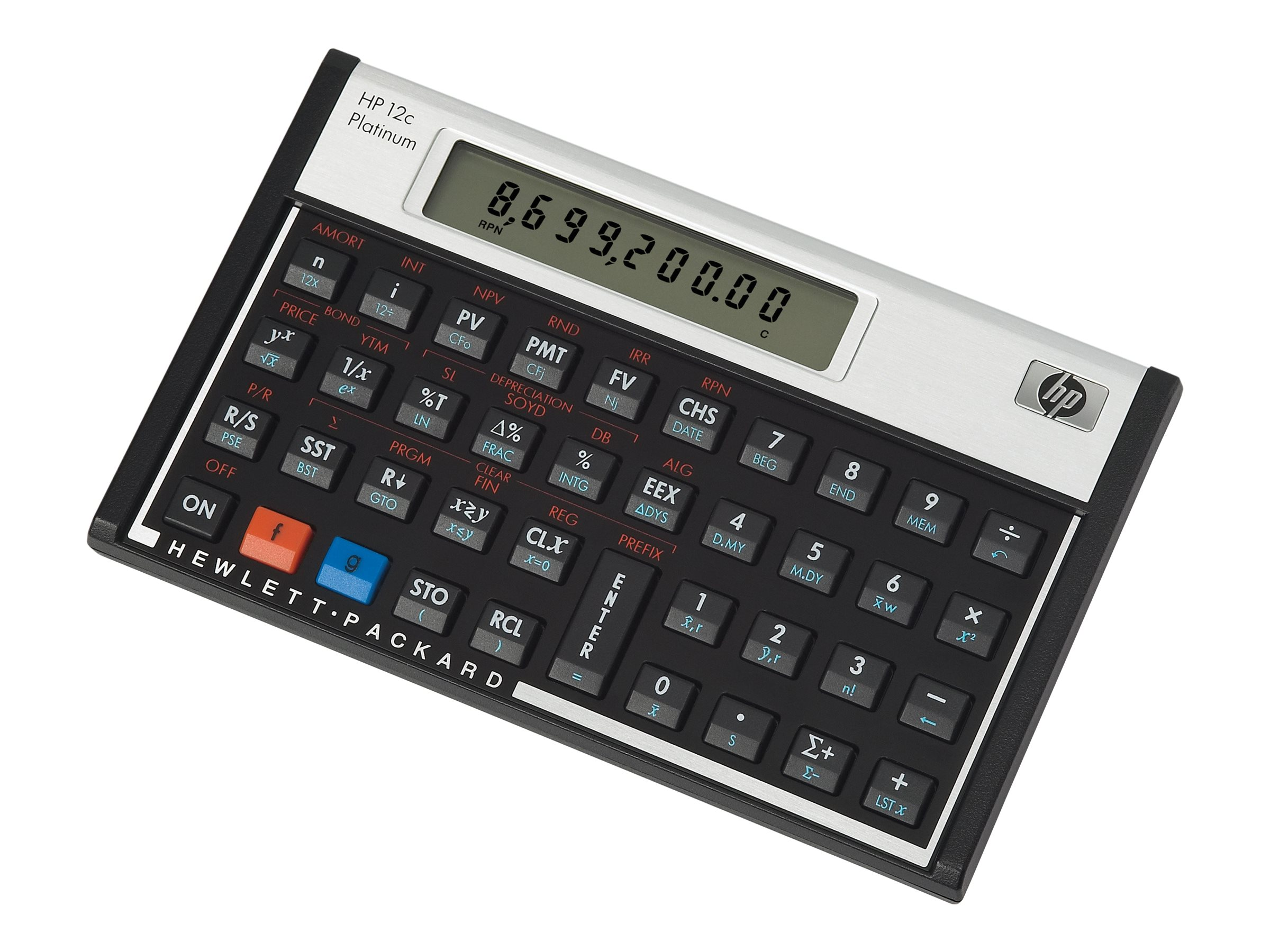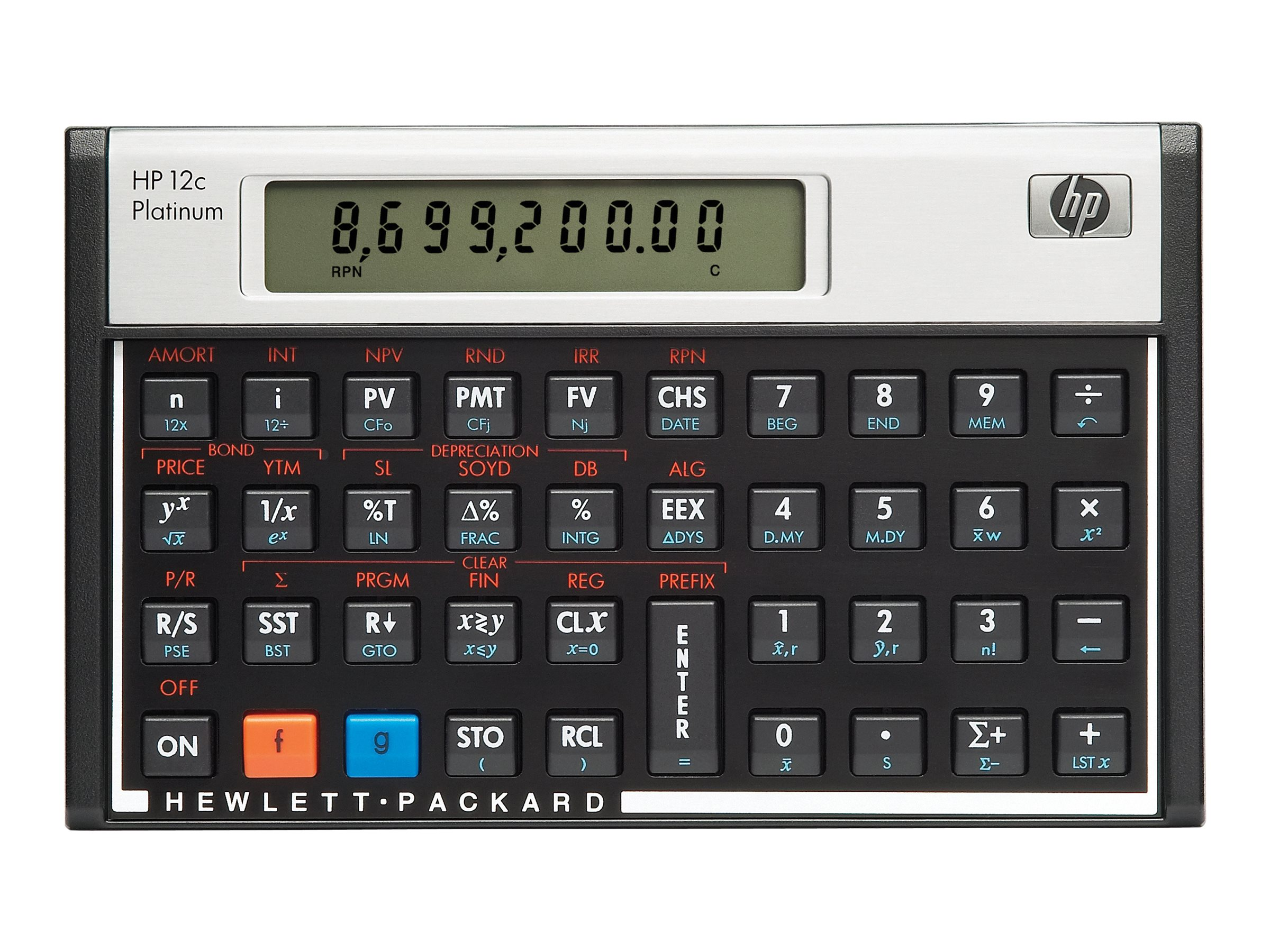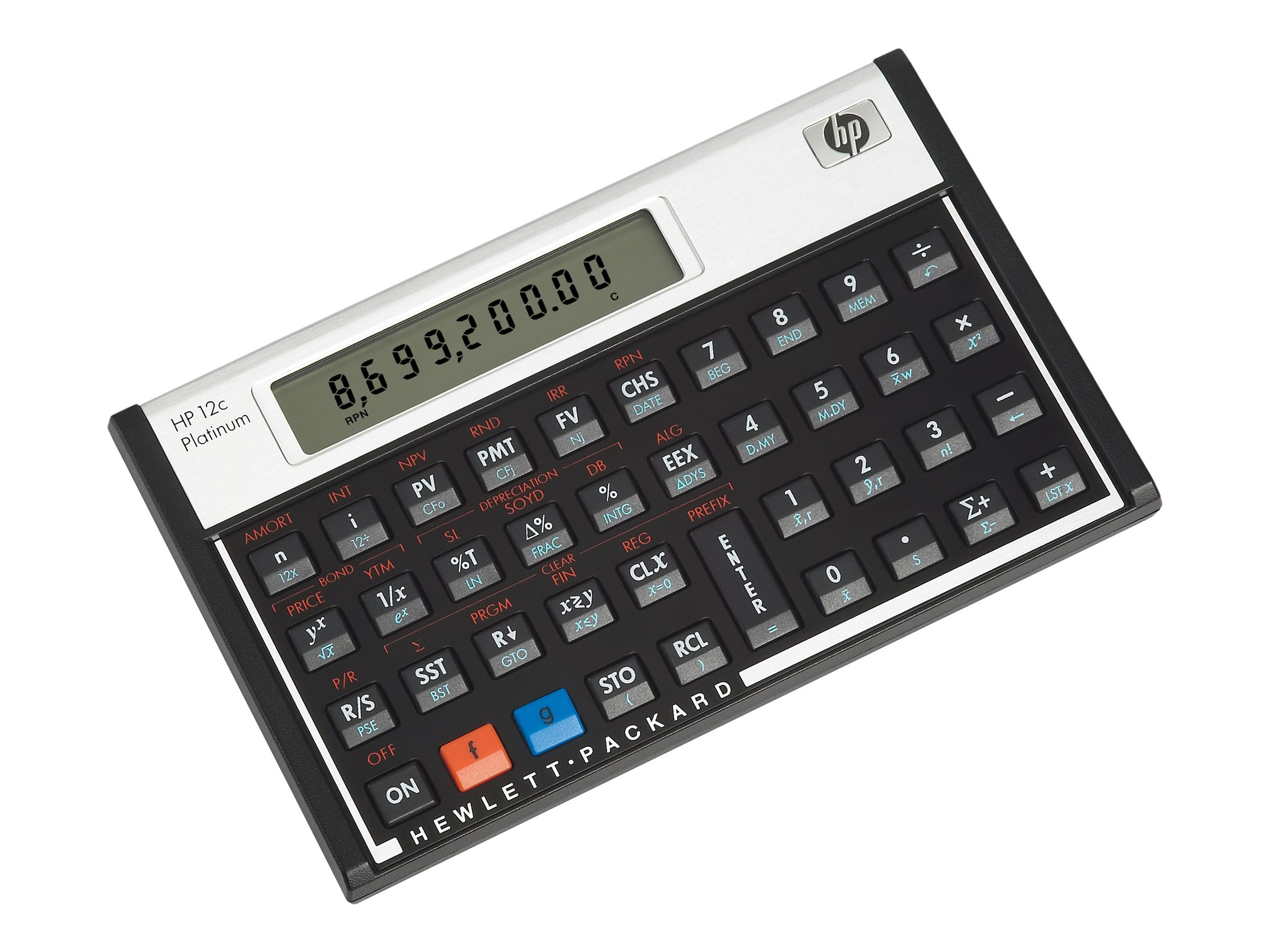# HP Inc. - HP 12c Platinum

Financial calculator - battery - silver, carbonite

••••HP Inc.
F2231AA#ABA
N/A
0883585518081
N/A

## Price: \$75.00

MSRP: \$79.99
Total Available: 0

## Specifications

Main Specifications
Product Description HP 12c Platinum - financial calculator
Product Type Financial calculator - LCD
Basic Functions Percent, sign change, backspace, time calculation, addition, subtraction, multiplication, division, date calculation, undo
Scientific Functions Rounding switch, square root, factorial, fraction, statistics, standard deviation, mean deviation, logarithm, exponent, parenthesis, linear regression, inverse, correlation deviation, weighted mean, internal precision (15 digits), powers, forecasting, Check and Correct (399 steps), integer, square
Financial Functions Loan, interest, cash change, interest conversion, amortization, cash flow, depreciation, Time Value of Money (TVM), savings, leasing, Internal Rate of Return (IRR), Net Present Value (NPV), Straight Line (SL), Sum-of-the-Year's Digits (SOYD), Declining Balance (DB), bonds
Display 1 line x 10 characters
Power Source Battery
Color Silver, carbonite
Features Auto power off, algebraic input mode, Reverse Polish Notation (RPN) input mode, 20 memory registers, Power off Memory Protection
General
Product Type Financial calculator
Power Source Battery
Features Auto power off, algebraic input mode, Reverse Polish Notation (RPN) input mode, 20 memory registers, Power off Memory Protection
Miscellaneous
Case Material Aluminum, plastic
Color Silver, carbonite
Included Accessories Batteries, carrying pouch
Warranty 1 year
Display
Type LCD - 1 line x 10 characters
Features Adjustable contrast
Dimensions & Weight
Width 5.1 in
Depth 3.1 in
Height 0.6 in
Weight 4.09 oz
Functions
Quantity 130
Basic Percent, sign change, backspace, time calculation, addition, subtraction, multiplication, division, date calculation, undo
Scientific Rounding switch, square root, factorial, fraction, statistics, standard deviation, mean deviation, logarithm, exponent, parenthesis, linear regression, inverse, correlation deviation, weighted mean, internal precision (15 digits), powers, forecasting, Check and Correct (399 steps), integer, square
Financial Loan, interest, cash change, interest conversion, amortization, cash flow, depreciation, Time Value of Money (TVM), savings, leasing, Internal Rate of Return (IRR), Net Present Value (NPV), Straight Line (SL), Sum-of-the-Year's Digits (SOYD), Declining Balance (DB), bonds
Battery Required
Form Factor CR2032
Required Qty 1
Run Time (Up To) 2 years

## Related Products

Below are the optional products related to the selected product.